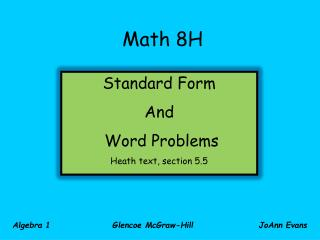DownloadDownload PresentationMath 8H

# Math 8H

Télécharger la présentation## Math 8H

- - - - - - - - - - - - - - - - - - - - - - - - - - - E N D - - - - - - - - - - - - - - - - - - - - - - - - - - -
##### Presentation Transcript

1. Standard Form And Word Problems Heath text, section 5.5 Math 8H Algebra 1 Glencoe McGraw-Hill JoAnn Evans

2. Slope-Intercept Form of a Linear Equation: y = mx + b Point-Slope Form of a Linear Equation: y – y1 = m(x – x1) Standard Form of a Linear Equation Ax + By = C Remember that in Standard Form, both variable terms are on the left side of the equal sign and the constant term is on the right side. Another feature of the Standard Form is that all coefficients are integers.

3. Write an equation in standard form of the line that passes through the point and has the given slope. Using y = mx + b

4. Candy corn costs \$2 per pound at the candy store and M&Ms cost \$3 per pound. With \$30 to spend, what are the different amounts of the two candies that you can buy? Let x = # pounds of M & Ms Let y = # pounds of candy corn Let 3x = VALUE of the M & Ms Let 2y = VALUE of the candy corn = + Value of the M & Ms Value of the candy corn Total Cost 3x + 2y = 30

5. Find the x- and y-intercepts. 16 14 3(0) + 2y = 30 y = 15 (all candy corn, no M & M’s) 12 10 Candy Corn (in pounds) 8 6 4 3x + 2(0) = 30 x = 10 (all M&Ms, no candy corn) 2 0 2 4 6 8 10 12 14 16 M & M’s (in pounds) Each point on the line represents a combination of the 2 candies that would have a total cost of \$30. Name some of the combinations.

6. Suppose you had \$6.00 to buy bananas and apples. Bananas cost \$0.49 per pound and apples cost \$0.34 per pound. Write a linear equation that represents the different amounts of fruit you could buy. Let x = weight of bananas Let y = weight of apples Let 49x = VALUE of bananas Let 34y = VALUE of apples Value of bananas Value of apples + = Total price 49x + 34y = 600

7. One possibility is that you could buy 10 pounds of bananas. How many pounds of apples would then be possible to buy? 49x + 34y = 600 49(10) + 34y = 600 490 + 34y = 600 -490 -490 34y = 110 y 3.2 lb of apples

8. You are running for class president and have \$48 to spend on publicity for your campaign. It costs \$2 to make a campaign button and \$1.20 to make a poster. Write an equation that represents the different numbers of buttons, x, and posters, y, that you could make. Let x = # of buttons Let y = # posters Let 2x = VALUE of the buttons Let 1.2y = VALUE of the posters + = Value of buttons Value of posters Total Cost 2x + 1.2y = 48 20x + 12y = 480 5x + 3y = 120

9. 40 x y 36 32 0 28 24 20 posters 16 12 8 4 0 4 8 12 16 20 24 28 32 36 40 buttons Should a line be drawn to connect the intercepts? Think for a minute to form an opinion. 24

10. This equation is in standard form. What can we learn by looking at it in slope-intercept form? x y 0 +3 -5 3 Look for this pattern in the table of values. The change in y is down 5; the change in x is up 3. y-intercept

11. 40 x y 36 32 0 +3 -5 28 3 24 6 20 posters 16 9 12 12 8 4 15 0 4 8 12 16 20 24 28 32 36 40 18 buttons 21 The slope you just found shows how the change in y and the change in x can help to find other possible combinations of buttons and posters. 24

12. Dogs sell for \$40 and cats sell for \$35 at Pets-R-Us. Sales figures for the busy holiday shopping season showed that the store received \$840 total for dog and cat sales in one weekend. Write an equation to describe the sales that weekend of dogs, x, and cats, y. Let x = # dogs Let y = # cats Let 40x = VALUE of dogs Let 35y = VALUE of cats + = Value of dogs Value of cats Total Cost 40x + 35y = 840 8x + 7y = 168

13. No! Who wants half a dog or two- thirds of a cat? x y 30 27 0 +7 -8 24 7 21 Should the x- and y-intercepts be connected in this case? 18 14 15 21 12 9 6 3 0 3 6 9 12 15 18 21 24 27 30 Put the equation in slope-intercept form. Using the slope information, find more combinations of dogs and cats.